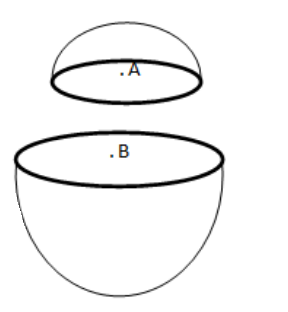# Solve the following :Question:

A thin spherical shell having uniform density is cut in two parts by a plane and kept separated as shown in figure (11-E3). The point $A$ is the centre of the plane section of the first part and B is the centre of the plane section of the second part. Show that the gravitational field at A due to the first part is equal in magnitude to the gravitational field at B due to the second part.Solution:

Resultant field inside any spherical shell is zero at all points. So, at point a and B, field is zero. The field due to upper part and lower part and are equal and opposite.